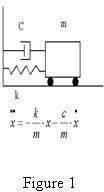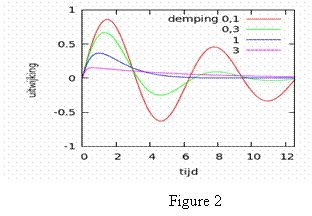# Do Engineers Use Differential Equations?

## Introduction

Instead of directly answering the question of "Do engineers use differential equations?" I would like to take you through some background first and then see whether differential equations are used by engineers.

Years ago when I was working as a design engineer for a shock absorber manufacturing company, my concern was how a hydraulic shock absorber dissipates shocks and vibrational energy exerted form road fluctuations to the car suspension system, how shock absorbers behave in the suspension system, and what are the effects of other elements of this system like the mass of the components, the spring rate of the suspension springs and tires, etc.

My research led me to a paper that used a very complicated nonlinear differential equation to study the functional behavior of hydraulic shock absorbers. Here I have to mention that in those days library and paper research was not as easy as today. Computers and the Internet were not as popular as today, and we had to search through engineering indexes which were annually published books containing abstracts of related published papers and articles and order the ones we wanted, if we could find any.

I am not going to discuss the nonlinear differential equation of hydraulics shock absorber here because it takes its own space and time and, more or less is beyond the scope of this article. All I can say is after reading that paper, I had a vivid idea about the function and behavior of shock absorbers in a suspension system.Later I used the Gauss-Jordan numerical method to solve differential equation of a damper-spring-mass system (figure 1) in a BASIC program. This way I had a simple simulation program by which I could not only understand the effects of different parameters of the system, but also feel the effects of changing, for instance, spring rate or damping coefficient. This simulating program enabled me to have direct practice of spring mass system as if I were working in a lab with real items. I cannot represent the actual results here because I have retired my old Amiga 500 computer which I used for that program with an up-to-date PC but, the results I got looked like figure 2.## So Do Engineers Use Differential Equations?

Simulations and simulators are hardware or software means to make one feel as if working in a real environment and gaining direct experience of a simulated subject system. Many engineering simulators use mathematical models of subject systems in the form of differential equations. Perhaps the reason why some engineers and engineering students feel differential equations are not used by engineers is that they are working with simulating and modeling software (such as the one shown in figure 3) and don’t see the actual mathematical model behind them.## What is a Differential Equation?

A differential equation is the relation between a function and its derivatives. Many engineering principles can be described based on such a relation. For instance in the damper-spring-mass system of figure 1:

x=f(t) is the unknown function of motion of the mass according to time t (independent variable)

-kx is the reactive force exerted by spring

dx/dt is change of distance according to change of time which is called velocity

-cdx/dt is the reactive force exerted by the damper

d2x/dt2 is change of velocity according to change of time which is called acceleration

md2x/dt2 is the force applied to the mass

Based on Newton’s Second Law of motion (F=ma)

md2x/dt2=-kx-cdx/dt or d2x/dt2=-(k/m)x-(c/m)dx/dt

## Summary

The objective of solving a differential equation is finding the unknown function (in the above example) known as the answer of the differential equation.This answer can be shown in analytical form, if applicable, or graphical form (figure 2 in above example) or any other visual form such as figure 4 for visualization of heat transfer in a pump casing, by solving the Heat equation.

Many scientific laws and engineering principles and systems are in the form of or can be described by differential equations. Differential equations are mathematical tools to model engineering systems such as hydraulic flow, heat transfer, level controller of a tank, vibration isolation, electrical circuits, etc.

Wherever it is possible to define a relation between a function and its derivatives, it is possible to make a mathematical model and study and understand the behavior of the system related to that function.